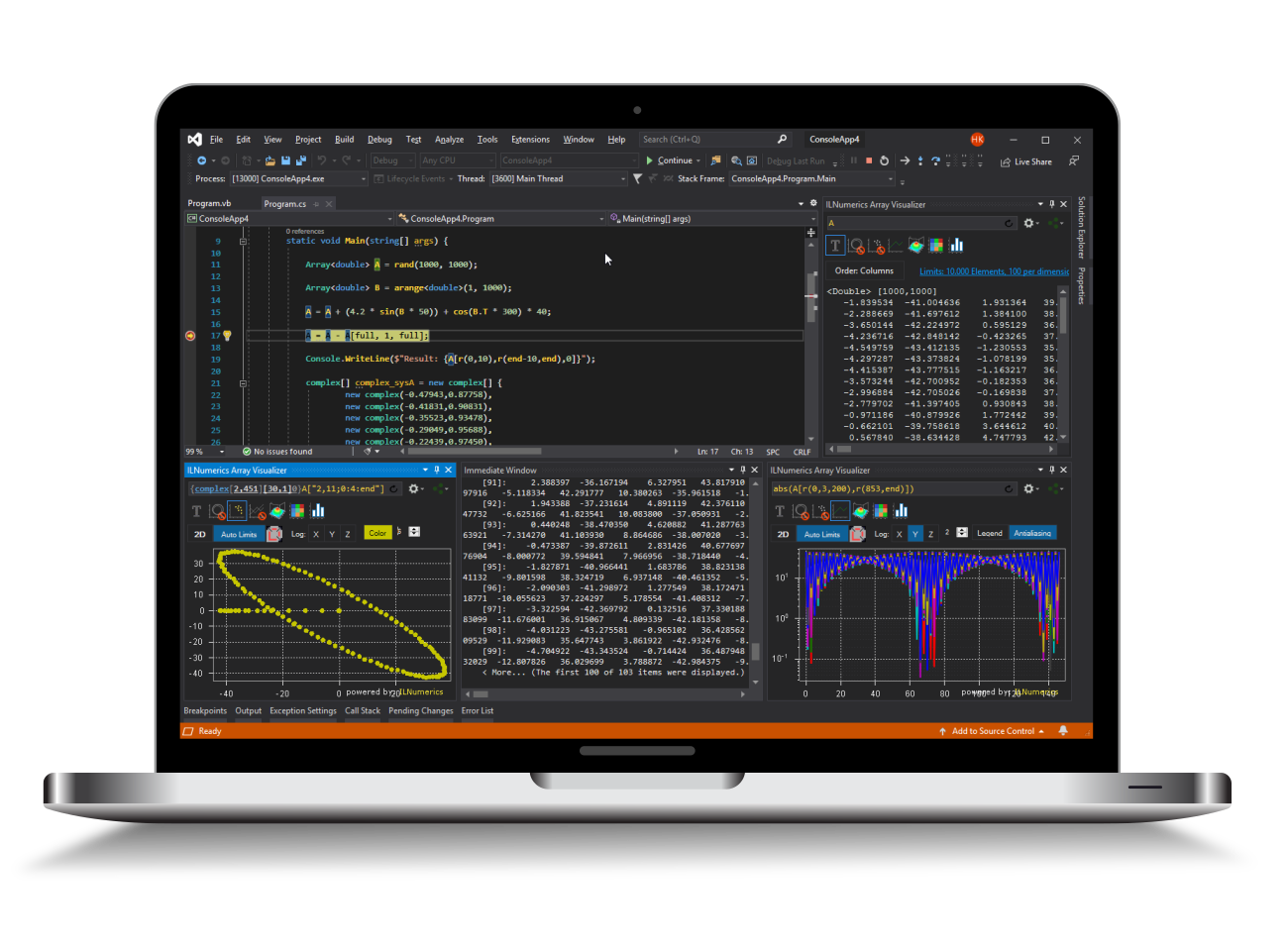Industrial Data Science
in C# and .NET:
Simple. Fast. Reliable.ILNumerics - Technical Computing

Modern High Performance Tools for Technical

Computing and Visualization in Industry and Science

# Array Operators

The following operators are defined on ILNumerics arrays. All operators work elementwise, are parallelized and may perform inplace on suitable arrays. Binary operators perform broadcasting according to the current setting of ILNumerics.Settings.ArrayStyle (see: Array Styles).

C# Operator Element type T returns

Function

equivalent

Description
Unary Operators - Array<T> A
Negation:  - all integer, double, float same as input

ILMath.

negate()

Negates the values of array elements. The result is the same as multiplying the values with -1. Some operators perform the negation with a larger data type than T. In this case the result is afterwards casted down to and stored as the datatype matching the input datatype.
Bitwise negation: ~ All integer types same as input

ILMath.

bitneg()

Reverses the bits of the array elements.
Explicit cast: (T) T (all types) System scalar -- Gives the System value T of the only element of a scalar Array<T>.
Implicit cast T (all types) Array<T> -- Instances of T (System.ValueType) implicitly converts to scalar Array<T> when required.
Indexing [ ] T (all types) Array<T> A.GetRange() A.SetRange() Indexing operator for subarray creation or assignment. See: indexing
Unary Operators - Logical A
NOT:  ! bool Logical

ILMath.

not()

Inverses the values of a logical array.
Explicit cast: (T) bool System scalar -- Gives the System.Boolean of the only element of a scalar Logical.
Implicit cast bool Logical -- System.Boolean is implicitly converted to a scalar Logical array when required.
Indexing [ ] bool Logical

L.GetRange()

L.SetRange()

Indexing operator for subarray creation or assignment. See: indexing
Binary Operators - Array<T> A, B
Arithmetic: +, -, *, /, % All numeric T, complex, fcomplex same as input

ILMath.

subtract()

multiplyElem()

divide()

mod();

subtract_sat()

multiplyElem_sat()

divide_sat()

mod_sat()

Elementwise arithmetic operations: plus, minus, multiply, division, modulo. The computation may be internally performed in a higher precision / larger integer type than T. The result, however, is stored into T elements. Overflows: if a result exceeds the natural limits of an integer datatype T, the behavior depends on the current ArrayStyle setting. numpy: no checks are done, 'wrapping around' may occur. ILNumericsV4: the result 'saturates' the limits of T, i.e.: values are clamped to the value range of datatype T. Integer operations are performed as 'double' floating point operations and rounded to the nearest integer afterwards.  See below for details.

Comparison:

==, !=, <,>,<=,>=

All numeric;

complex, fcomplex where applicable

Logical

ILMath.

eq()

neq()

lt()

gt()

le()

ge()

Elementwise comparison operators: equal to, unequal to, lower [or equal], greater [or equal]. The result of the comparison is returned at the corresponding element position in a Logical array.

Special floating point values: comparisons of two NaN values gives false.

(Use eqnan() for comparing arrays with expected NaN values.)

Bitwise:

&, |, ^, <<, >>

All integer same as input

ILMath.

bitand()

bitor()

bitxor()

lshift()

rshift()

Bitwise operators: and, or, xor. Bit shifting operators << and >> require an integer value as second operand.
Binary Operators - Logical A,B

Comparison:

==, !=

bool Logical

ILMath.

eq()

neq()

Elementwise comparison operators: equal to, unequal to.

Logical:

&, |, ^

bool Logical

ILMath.

and()

or()

xor()

Elementwise logical operators: logical and, logical or, logical xor.

All operations involving two arrays are performed with broadcasting: singleton dimensions of the one array are expanded by (virtual) replication to match the size of the corresponding dimension of the other array. If both arrays have different number of dimensions, the dimensions are aligned at the first dimension (Matlab array style) or the last dimension (numpy array style) and missing trailing / leading dimensions of the one array with fewer dimensions are (virtually) replaced by singleton dimensions.

Broadcasting is a performance feature, potentially saving many memory copies. It is available in ILNumerics since version 4.10, in Matlab and numpy.

## Static Functions

For most operators corresponding static functions are provided on the ILMath class. Use the static function directly when using an operator is not supported in your language.

## Integer Operations: Saturation, Rounding

Some operators may perform saturation (Matlab style on integer arrays). Internally, a corresponding saturating function is called depending on the current array style. The names of saturate-and-round functions end with "[name]_sat". Here, [name] is the name of the main static function: add(), add_sat(), subtract(), subtract_sat(),etc. Thus, by using the static functions directly, one can override the array style without having to switch between individual array style settings:

## Operator Precedence

If multiple operators are combined in a single expression, operators take precedence according to the following list (starting with the highest precedence):

• [ ] indexing
• () casting
• -, !, ~  unary negation
• *, /, % - multiplicative arithmetic
• +, -  addition & subtraction
• <<, >> bit shifts
• <,>,<=,>= relational comparison
• ==, != equality comparison
• &, ^, | - logical / bitwise and / xor / or (in this order)

Being a DSL, ILNumerics array operators rely on the operator precedence of the host language.